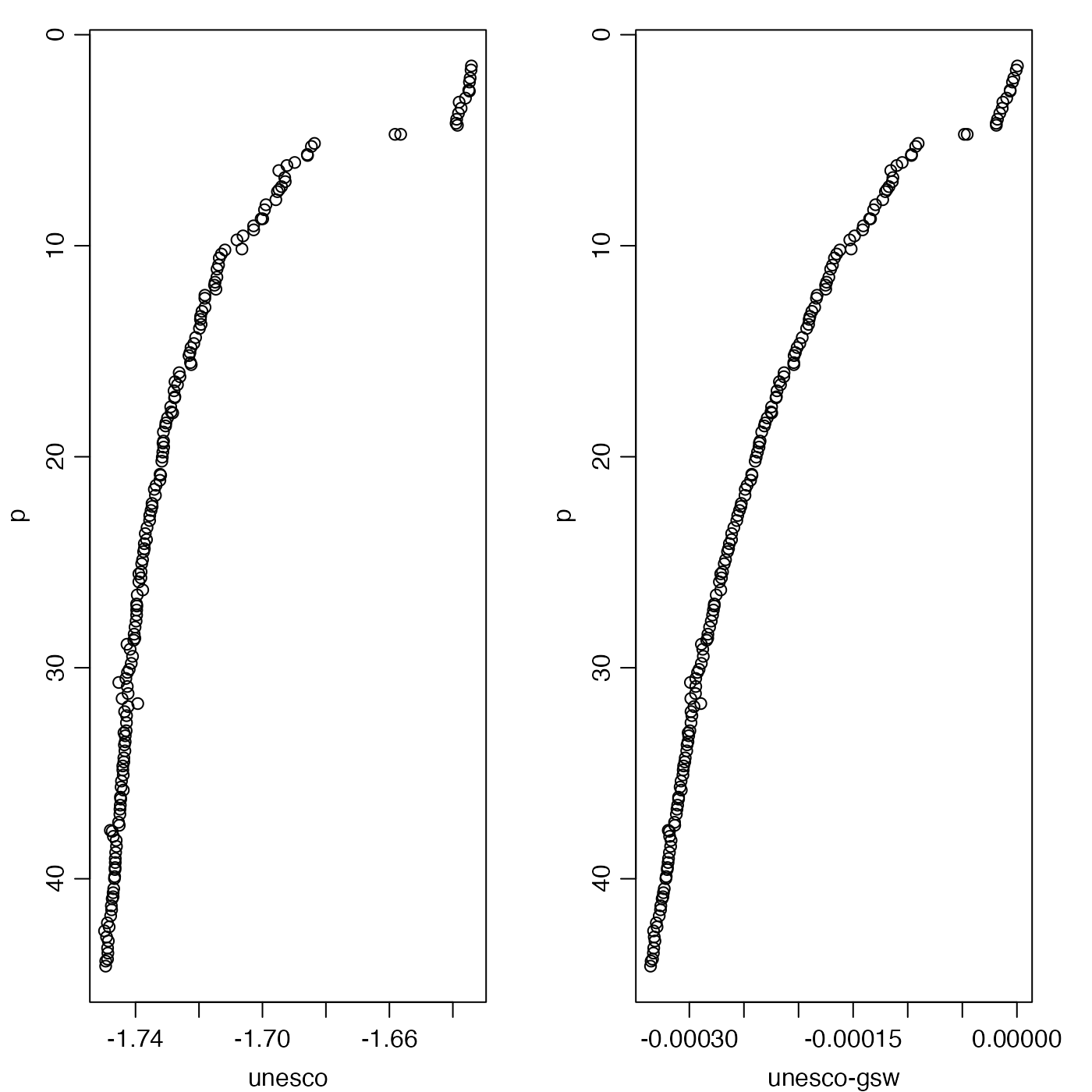Compute in-situ freezing temperature of seawater, using either the UNESCO formulation (computed as in Section 5 of Fofonoff and Millard, 1983) or the GSW formulation (computed by using gsw::gsw_SA_from_SP() to get Absolute Salinity, and then gsw::gsw_t_freezing() to get the freezing temperature).

swTFreeze(
salinity,
pressure = NULL,
longitude = NULL,
latitude = NULL,
saturation_fraction = 1,
eos = getOption("oceEOS", default = "gsw")
)

## Arguments

salinity

Either practical salinity (PSU) or a ctd object from which practical salinity and pressure (plus in the eos="gsw" case, longitude and latitude) are inferred.

pressure

Seawater pressure (dbar).

longitude

Longitude of observation (only used if eos="gsw"; see “Details”).

latitude

Latitude of observation (only used if eos="gsw"; see “Details”).

saturation_fraction

The saturation fraction of dissolved air in seawater, ignored if eos="unesco").

eos

The equation of state, either "unesco" (Fofonoff and Millard, 1983; Gill 1982) or "gsw" (IOC, SCOR and IAPSO 2010; McDougall and Barker 2011).

## Value

Temperature (degC), defined on the ITS-90 scale.

## Details

If the first argument is an oce object, and if the pressure argument is NULL, then the pressure is sought within the first argument. In the case of eos="gsw", then a similar procedure also applies to the longitude and latitude arguments.

Other functions that calculate seawater properties: T68fromT90(), T90fromT48(), T90fromT68(), computableWaterProperties(), locationForGsw(), swAbsoluteSalinity(), swAlphaOverBeta(), swAlpha(), swBeta(), swCSTp(), swConservativeTemperature(), swDepth(), swDynamicHeight(), swLapseRate(), swN2(), swPressure(), swRho(), swRrho(), swSCTp(), swSR(), swSTrho(), swSigma0(), swSigma1(), swSigma2(), swSigma3(), swSigma4(), swSigmaTheta(), swSigmaT(), swSigma(), swSoundAbsorption(), swSoundSpeed(), swSpecificHeat(), swSpice(), swSstar(), swTSrho(), swThermalConductivity(), swTheta(), swViscosity(), swZ()

Dan Kelley

## Examples

# 1. Test for a check-value given in reference 1. This value, -2.588567 degC,
# is in the 1968 temperature scale (IPTS-68), but swTFreeze reports
# in the newer ITS-90 scale, so we must convert before checking.
Tcheck <- -2.588567 # IPTS-68
T <- swTFreeze(salinity=40, pressure=500, eos="unesco")
stopifnot(abs(Tcheck - T68fromT90(T)) < 1e-6)

# 2. Compare unesco and gsw formulations.
data(ctd)
p <- ctd[["pressure"]]
par(mfrow=c(1, 2), mar=c(3, 3, 1, 2), mgp=c(2, 0.7, 0))
plot(swTFreeze(ctd, eos="unesco"),
p, xlab="unesco", ylim=rev(range(p)))
plot(swTFreeze(ctd, eos="unesco") - swTFreeze(ctd, eos="gsw"),
p, xlab="unesco-gsw", ylim=rev(range(p)))